Next: Implementation Up: Optimization Previous: Definition of a minimum   Contents

Methods

A first method to find all the solutions of an optimum problem is to consider the system of derivative equations and find its root (eventually under the given constraints): this may be done with the solving procedures described in a previous chapter. Hence we have implemented an alternative method which is able to work even if the optimum function has, globally or locally, no derivatives. This method is implemented as a special case of the general solving procedures. The only difference is that the procedure maintains a value for the current optimum: during the bisection process we evaluate the optimum function for each new box and reject those that are not compatible with the current optimum.

Two types of method enable to solve an optimization problem:

1. a method which need only a procedure for evaluating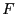,
2. a method which need a procedure for evaluatingand a procedure for evaluating its gradient,
Note that with the first method none of the function needs to be differentiable while for the other one not all the functions in the set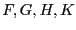must be differentiable: only one function in the set has to be differentiable.

For the first method we update the current optimum by computing the optimum function value at the middle point of the box. For the method with the gradient a local optimizer based on the steepest descent method is also used if there is only one equation to be minimized or if there are only inequalities constraints. The local optimizer works in 2 steps: first a rough optimization and then a more elaborate procedure if the result of the first step is better than a fixed threshold defined by the global variable ALIAS_Threshold_Optimiser whose default value is 100. Additionally if an extremum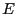has been already determined the local optimizer (which may computer intensive) is called only if the function value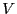at the middle point is such that for a maximum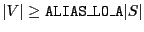and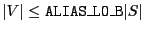for a minimum, where ALIAS_LO_A and ALIAS_LO_B are global variables with default value 0.9 and 1.1.Next: Implementation Up: Optimization Previous: Definition of a minimum   Contents
Jean-Pierre Merlet 2012-12-20